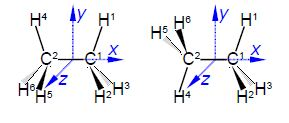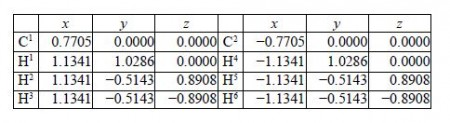Chemistry

# Sample Questopn: Methane Molecular Structure Analysis

Using the coordinate data from the solution to Question “Methane Molecular Structure Analysis, and so find the coordinates for the carbon and hydrogen atoms in ethane for the eclipsed and staggered conformations. The C-C bond length is 1.541 A and C-H bond distance is 1.091 A as above and all bond angles are tetrahedraL You may find it helpful in each case to make a molecular model and consider the symmetry relationships between corresponding atoms in each of the structures.Solution

The C-C bond length is 1.541 A and C-H bond length is 1.091 A and all bond angles are tetrahedral 109.47°. For the eclipsed conformation the coordinates of C1, H1, H2, and H3 are similar to methane except the x coordinates are increased by 1.541 A/2 = 0.7705 A. The coordinates of C2, H4, H5, and H1 are related to C1, H1, FP, and H3 by reflection in the mirror in the yz plane i.e. the x coordinates change sign.The coordinates of C1, C2, H1, H2, and H3 of the staggered conformation of ethane are identical to those above in above Figure. The coordinates of H4, H5, and H6 are related to H1, H2, and H3 by inversion through the centre of symmetry, i.e. the coordinates of H4, H5, and H6 are the same as H1, H2, and H3 but with an inversion of their sign.